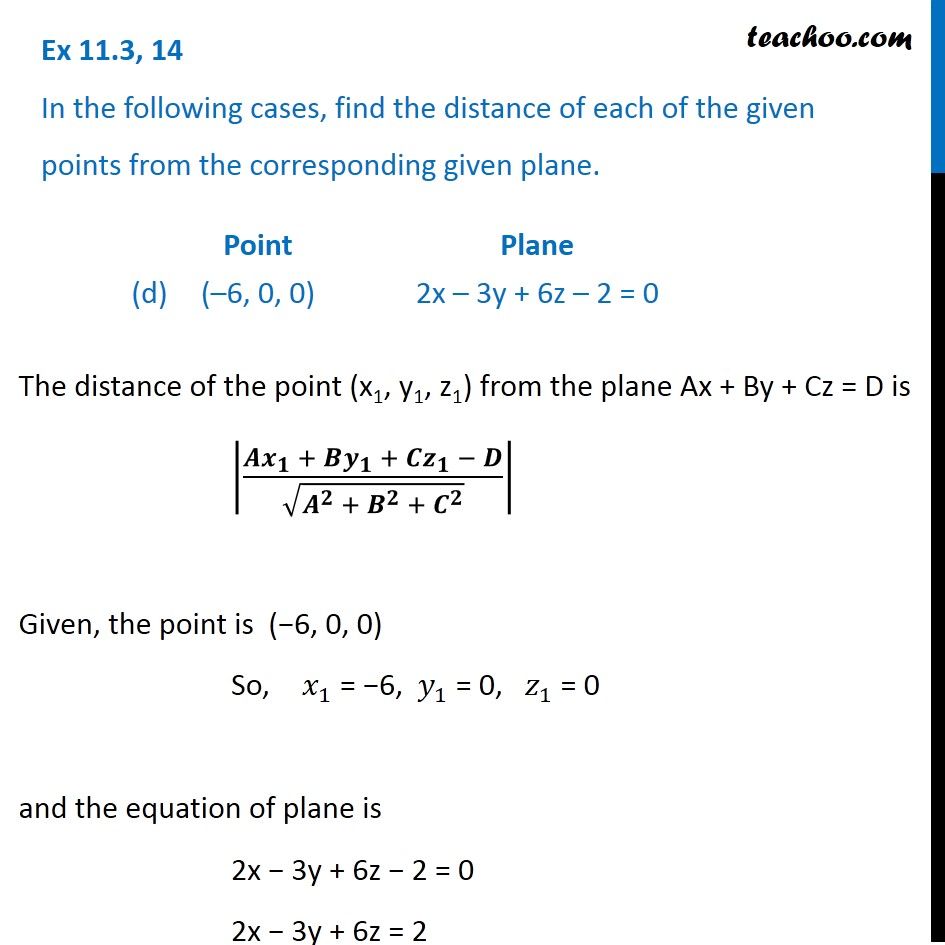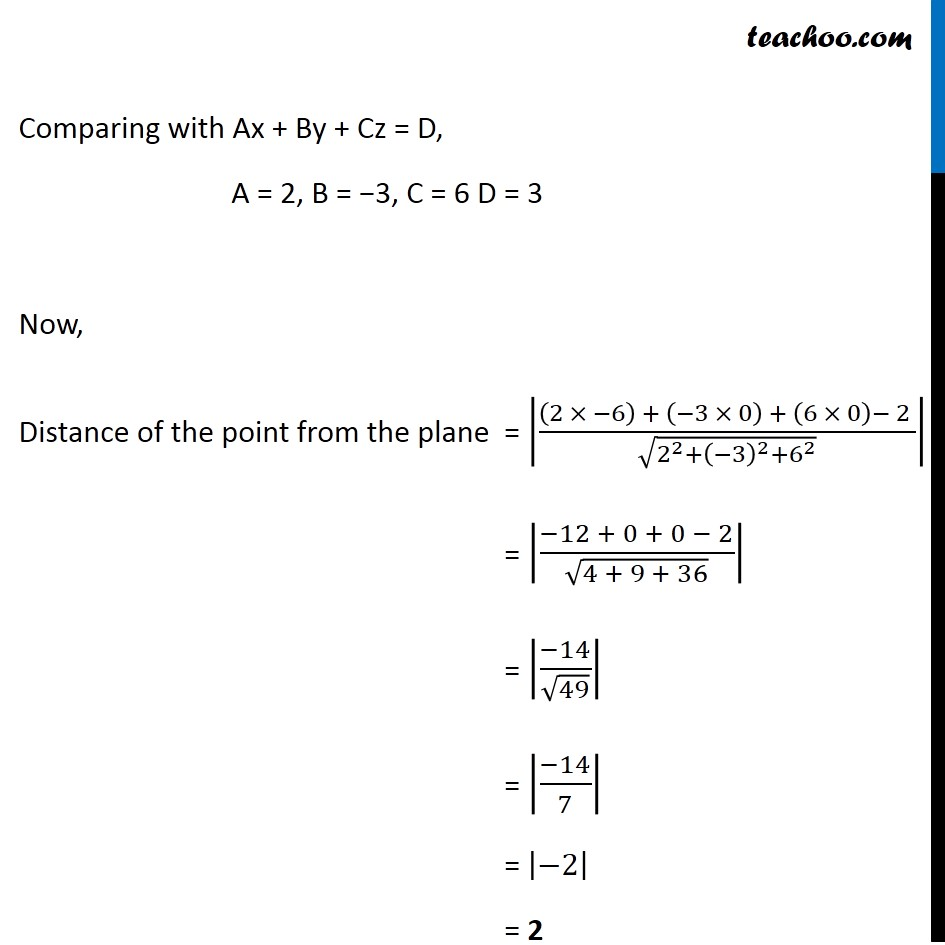Plane

Chapter 11 Class 12 Three Dimensional Geometry
Serial order wiseLearn in your speed, with individual attention - Teachoo Maths 1-on-1 Class

### Transcript

Question 14 In the following cases, find the distance of each of the given points from the corresponding given plane. The distance of the point (x1, y1, z1) from the plane Ax + By + Cz = D is |(𝑨𝒙_𝟏 + 〖𝑩𝒚〗_𝟏 +〖 𝑪𝒛〗_𝟏 − 𝑫)/√(𝑨^𝟐 + 𝑩^𝟐 + 𝑪^𝟐 )| Given, the point is (−6, 0, 0) So, 𝑥_1 = −6, 𝑦_1 = 0, 𝑧_1 = 0 and the equation of plane is 2x − 3y + 6z − 2 = 0 2x − 3y + 6z = 2 Comparing with Ax + By + Cz = D, A = 2, B = −3, C = 6 D = 3 Now, Distance of the point from the plane = |((2 × −6) + (−3 × 0) + (6 × 0)− 2 )/√(2^2+(−3)^2+6^2 )| = |(−12 + 0 + 0 − 2)/√(4 + 9 + 36)| = |(−14)/√49| = |(−14)/7| = |−2| = 2